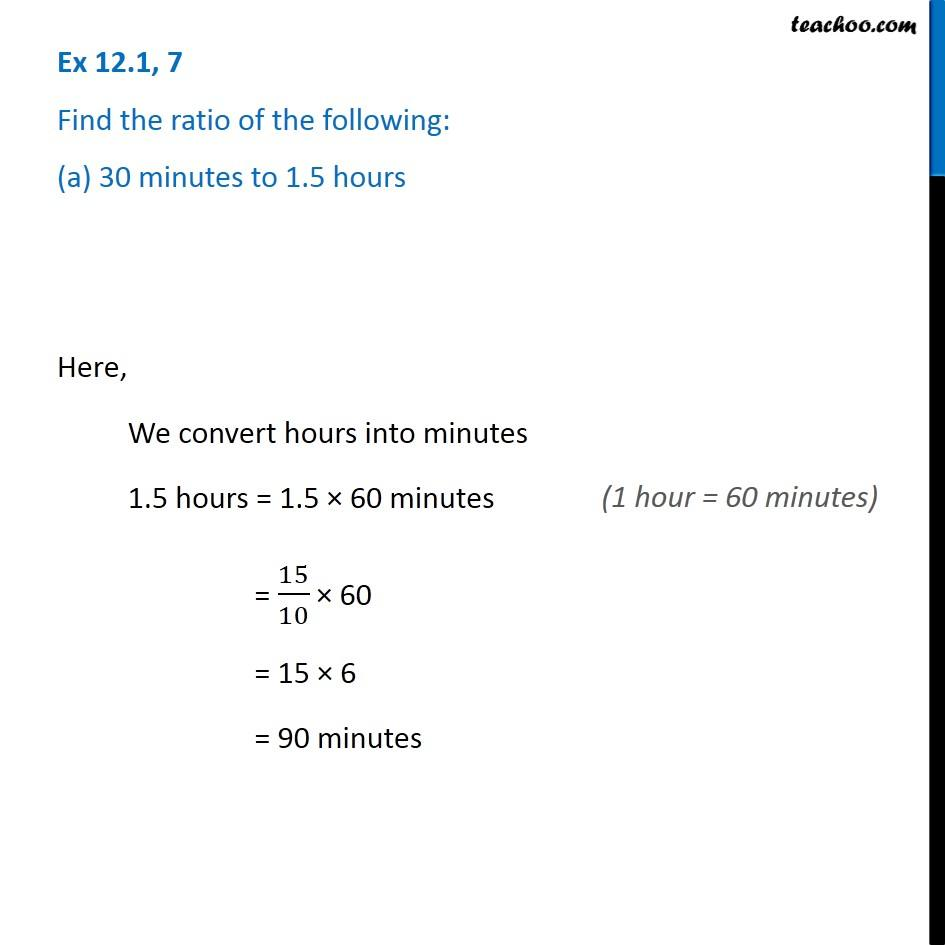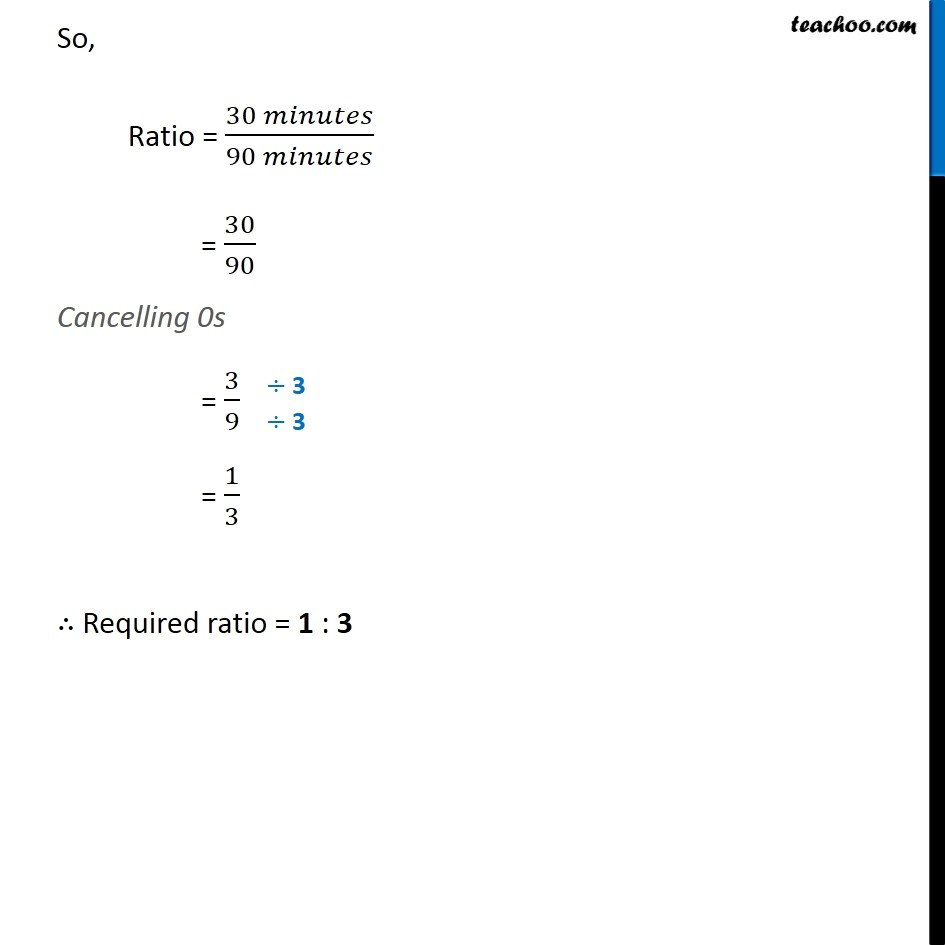Finding ratio by changing units

Chapter 12 Class 6 Ratio And Proportion
Concept wiseIntroducing your new favourite teacher - Teachoo Black, at only ₹83 per month

### Transcript

Ex 12.1, 7 Find the ratio of the following: (a) 30 minutes to 1.5 hours Here, We convert hours into minutes 1.5 hours = 1.5 × 60 minutes = 15/10 × 60 = 15 × 6 = 90 minutes So, Ratio = (30 𝑚𝑖𝑛𝑢𝑡𝑒𝑠)/(90 𝑚𝑖𝑛𝑢𝑡𝑒𝑠) = 30/90 Cancelling 0s = 3/9 = 1/3 ∴ Required ratio = 1 : 3